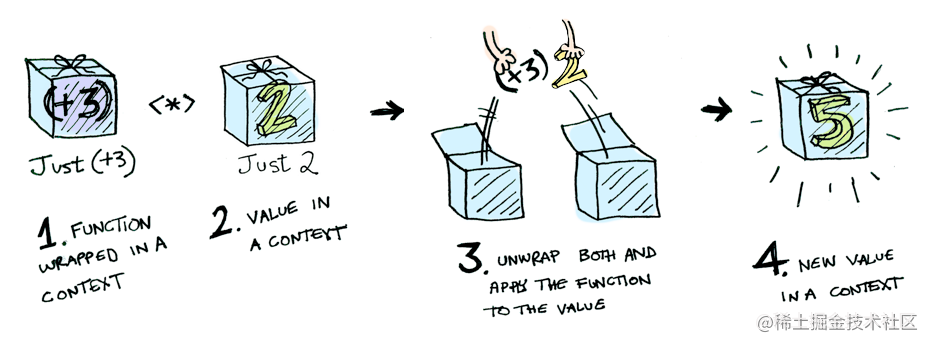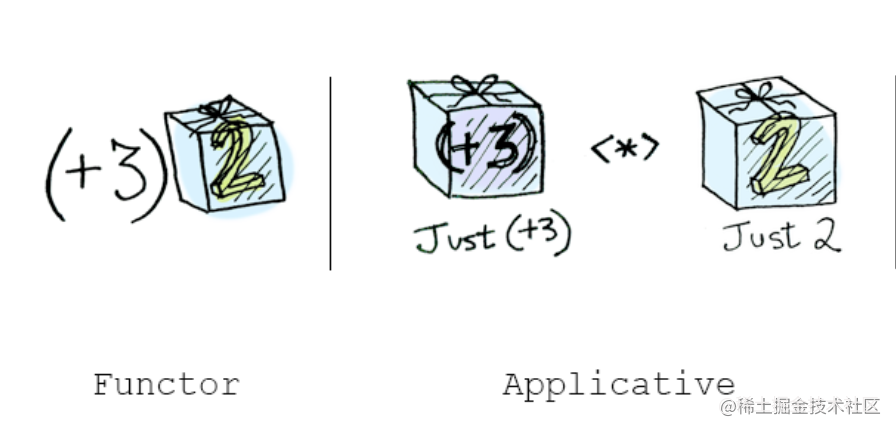# 函数式编程进阶：应用函子网易云音乐

### 纯函数与副作用

• 给出相同的参数，那么函数的返回值一定相同。该函数结果值不依赖任何隐藏信息或程序执行处理可能改变的状态，也不能依赖于任何来自 I/O 的外部输入。
• 在对函数返回值的计算过程中，不会产生任何语义上可观察的副作用或输出，例如对象的变化或者输出到 I/O 的操作。

### 惰性盒子-LazyBox

JavaScript 函数具有值的行为，也就是说，函数就是一个基于输入的且尚未求值的不可变的值，或者可以认为函数本身就是一个等待计算的惰性的值。那么我们完全可以把这个“惰性的值”装入 `Box` 中，然后延迟调用即可，仿照上一章的 `Box` ，可以实现一个 `Lazy Box`

``````const LazyBox = g => ({
map: f => LazyBox(() => f(g())),
fold: f => f(g())
})

``````const finalPrice = str =>
LazyBox(() => str)
.map(x => { console.log('str:', str); return x })
.map(x => x * 2)
.map(x => x * 0.8)
.map(x => x - 50)

const res = finalPrice(100)
console.log(res)  // => { map: [Function: map], fold: [Function: fold] }

``````const app = finalPrice(100)
const res2 = app.fold(x => x)

console.log(res2) // => 110

`fold` 函数就像打开潘多拉魔盒的双手；通过 `LazyBox` 我们把可能会“弄脏双手(产生副作用)”的代码扔给了最后的 `fold` ，这样做又有什么意义呢？

• 把代码中不纯的部分剥离出来，保障核心部分代码的 “pure” 特性，比如上面的代码中只有 `app.fold(x => x)` 是 “no pure” 的，其他部分都是 “pure”
• 类似于上一章中的错误集中管理，可以通过 `LazyBox` 来把副作用集中管理，如果在项目中不断的扩大 “pure” 的部分，我们甚至可以把不纯的代码推到代码的边缘，保证核心部分的 “pure” 和 “referential transparency”

LazyBox 也和 Rxjs 中的 `Observable` 有很多相似之处，两者都是惰性的，在 `subscribe` 之前，`Observable` 也不会推送数据。

### 应用函子

#### Function in Box

``````const Box = x => ({
map: f => Box(f(x)),
inspect: () => `Box(\${x})`
})

const addOne = x => x + 1
Box(addOne) // => Box(x => x + 1)

`inspect` 方法的目的是为了使用 Node.js 中的 `console.log` 隐式的调用它，方便我们查看数据的类型；而这一方法在浏览器中不可行，可以用 `console.log(String(x))` 来代替; Node.js V12 API 有变更，可以采用 `Symbol.for('nodejs.util.inspect.custom')` 替代 `inspect`

``````const Box = x => ({
map: f => Box(f(x)),
apply: o => o.map(x),
flod: f => f(x),
inspect: () => `Box(\${x})`
})``````F(x).map(f) == F(f).ap(F(x))

`Applicative functor (应用函子)` 也是函数式编程中一大堆“故弄玄虚”的概念中唯一的比较“名副其实”的了，想想 `Functor(函子)`

#### 应用函子与函数柯里化

``````const add = (x, y) => x + y
const curriedAdd = x => y => x + y

``````const add = x => y => x + y

``````Box(add).ap(Box(1))  // => Box(y => 1 + y) (得到另一个应用函子)

### 应用函子的应用案例

``````const checkUserInfo = user => {
const { name, pw, phone } = user
const errInfo = []
if (/^[0-9].+\$/.test(name)) {
errInfo.push('用户名不能以数字开头')
}
if (pw.length <= 6) {
errInfo.push('密码长度必须大于6位')
}

if (errInfo.length) {
return errInfo
}
return true
}

const userInfo = {
name: '1Melo',
pw: '123456'
}

const checkRes = checkUserInfo(userInfo)
console.log(checkRes)  // => [ '用户名不能以数字开头', '密码长度必须大于6位' ]

``````const Right = x => ({
x,
map: f => Right(f(x)),
ap: o => o.isLeft ? o : o.map(x),
fold: (f, g) => g(x),
isLeft: false,
isRight: true,
inspect: () => `Right(\${x})`
})

const Left = x => ({
x,
map: f => Left(x),
ap: o => o.isLeft ? Left(x.concat(o.x)) : Left(x),
fold: (f, g) => f(x),
isLeft: true,
isRight: false,
inspect: () => `Left(\${x})`
})

`Left` 分支上的 `ap: o => o.Left ? Left(x.concat(o.x)) : Left(x)`，如果是 `Left` 的实例，则进行一个“叠加”，实际上就是为了累加错误信息，而如果不是 `Left` 的实例则直接返回原本已经记录的错误信息。

``````const checkName = name => {
return /^[0-9].+\$/.test(name) ? Left('用户名不能以数字开头') : Right(true)
}

const checkPW = pw => {
return pw.length <= 6 ? Left('密码长度必须大于6位') : Right(true)
}

``````const R = require('ramda')

const success = () => true

function checkUserInfo(user) {
const { name, pw, phone } = user
// 2 是因为我们需要 `ap` 2 次。
const returnSuccess = R.curryN(2, success);

return Right(returnSuccess)
.ap(checkName(name))
.ap(checkPW(pw))
}

const checkRes = checkUserInfo({ name: '1Melo', pw: '123456' })
console.log(checkRes) // => Left(用户名不能以数字开头密码长度必须大于6位)

const checkRes2 = checkUserInfo({ name: 'Melo', pw: '1234567' })
console.log(checkRes2) // => Right(true)

### PointFree风格

``````const apply2 = (T, g, funtor1, functor2) => T(g).ap(funtor1).ap(functor2)

function checkUserInfo(user) {
const { name, pw, phone } = user
const returnSuccess = R.curryN(2, success);

return apply2(Right, returnSuccess, checkName(name), checkPW(pw))
}

`apply2` 函数的参数特别多，尤其是需要传递 `T` 这个不确定的容器，用来把普通函数 `g` 装进盒子里。

``````F(f).ap(F(x)) == F(x).map(f)

``````const apply2 = (T, g, funtor1, functor2) => T(g).ap(funtor1).ap(functor2)

const liftA2 = (g, funtor1, functor2) => funtor1.map(g).ap(functor2)

``````function checkUserInfo(user) {
const { name, pw, phone } = user
const returnSuccess = R.curryN(2, success);

return liftA2(returnSuccess, checkName(name), checkPW(pw))
}

``````const liftA3 = (g, funtor1, functor2, functor3) => funtor1.map(g).ap(functor2).ap(functor3)
const liftA4 = (g, funtor1, functor2, functor3, functor4) => funtor1.map(g).ap(functor2).ap(functor3).ap(functor4)

### Applicative Functor 和 Functor 的区别和联系

• Functor: 应用一个函数到包裹的值：`Box(1).map(x => x+1)`.
• Applicative: 应用一个包裹的函数到包裹的值：`Box(x => x+1).ap(Box(1))`